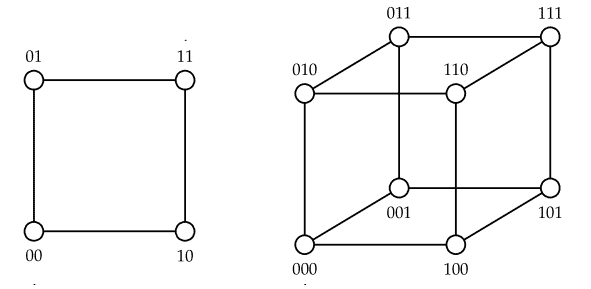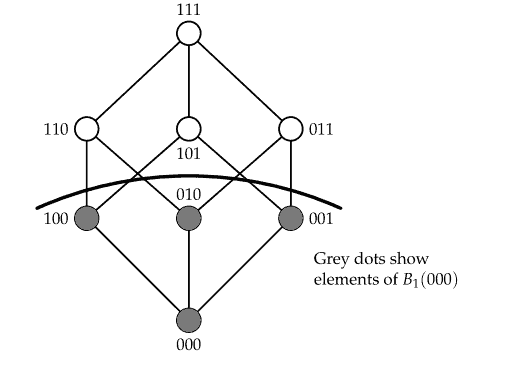## Hamming distance and error detection and correction

We define Hamming distance and use it to give a precise statement of what it means to decode by choosing the ‘closest codeword’ to a received word. We will also see some more examples of codes used for error detection and correction.

You may be able to guess the definition of Hamming distance (for binary words) from the diagrams showing ${0,1}^{2}$ and ${0,1}^{3}$ below.${0,1}^{2}$ and ${0,1}^{3}$

We will use Hamming distance to make precise our intuitive idea that a code has a maximum number of errors it can hope to detect and correct reliably, and see different ways to calculate this number.

Definition Let $A$ be an alphabet. Let $u, v \in A^{n}$ be words of length $n$. The Hamming distance between $u$ and $v$, denoted $d(u, v)$, is the number of positions in which $u$ and $v$ are different.

In mathematical notation, $d(u, v)=\left|\left{i \in{1,2, \ldots, n}: u_{i} \neq v_{i}\right}\right| .$ We will often abbreviate ‘Hamming distance’ to ‘distance’.

Example Working with binary words of length 4, we have
$$d(0011,1101)=3$$
because the words 0011 and 1101 differ in their first three positions, and are the same in their final position. Working with words over the 26 -ary alphabet ${\mathrm{A}, \mathrm{B}, \mathrm{C}, \ldots, \mathrm{X}, \mathrm{Y}, \mathrm{Z}}$ we have $d(\mathrm{TALE}, \mathrm{TAKE})=1$ and $d(\mathrm{TALE}, \mathrm{TILT})=2$
$\square$

Exercise Check that $d(1010,1001)=2 .$ Find the number of binary words $v$ of length 4 such that $d(1010, v)=r$ for each $r \in{0,1,2,3,4}$. Do you recognize the sequence you get?
The next theorem shows that Hamming distance has the expected properties of a distance. Part (iii) is the triangle inequality for Hamming distance: it will be used in Sections 3 and 4 later.
Theorem 2.4. Let $A$ be a $q$ -ary alphabet and let $u, v, w$ be words over $A$ of length $n$.
(1) $d(u, v)=0$ if and only if $u=v$;
(2) $d(u, v)=d(v, u)$
(3) $d(u, w) \leq d(u, v)+d(v, w)$.

## HAMMING DISTANCE AND NEAREST NEIGHBOUR DECODINGGrey dots show elements of $B_{1}(000)$

Using Hamming distance we can define Hamming ball of radius $t$ about a word $u$, consisting of all the words that can be obtained from $u$ by changing up to $t$ of its positions.

Definition Let $A$ be a $q$ -ary alphabet and let $u$ be a word of length $n$. The Hamming ball of radius $t$ about $u$ is
$$B_{t}(u)=\left{v \in A^{n}: d(u, v) \leq t\right} .$$
The diagram below shows the Hamming ball of radius 1 about 000 .

Example The Hamming balls about the binary word 0000 are
\begin{aligned} &B_{0}(0000)={0000} \ &B_{1}(0000)={0000,1000,0100,0010,0001}, \ &B_{2}(0000)=B_{1}(0000) \cup{1100,1010,1001,0110,0101,0011} \ &B_{3}(0000)=B_{2}(0000) \cup{1110,1101,1011,0111} \end{aligned}
and $B_{4}(0000)$ is the set of all binary words of length $4 .$ The Hamming balls about the binary word 1010 are
\begin{aligned} B_{0}(1010) &={1010} \ B_{1}(1010) &={1010,0010,1110,1000,1011} \ B_{2}(1010) &=B_{1}(1010) \cup{0110,0000,0011,1100,1111,1001} \end{aligned}
Also $B_{3}(1010)$ consists of all binary words of length 4 except 0101 , and $B_{4}(1010)$ is the set of all binary words of length 4 \square
We will see later how Hamming balls are used to prove important results about error correcting codes.

The decoded message is correct if and only if $w=u$. This is the case if and only if $u$ is the unique nearest codeword to $v$, i.e.
$$d(u, v)<d\left(u^{\prime}, v\right)$$
for all codewords $u^{\prime}$ different from $u .$
given a received word $v$ we decode it to the codeword $u$ such that $\mathbf{P}(u$ sent $\mid v$ received $)$ is maximised, that is, we decode $v$ to the codeword $u$ most likely to have resulted in $v$. This also seems an intuitively obvious way to decode. However, you can find simple examples to show that the two methods do not always decode to the same codeword. Theorem states when the two methods coincide for the binary symmetric channel.